# 4D ROTATION AND SPHERE PACKING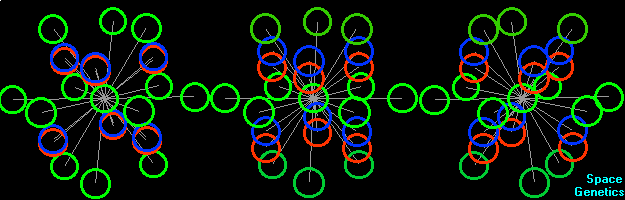4-d rotation.
It's beatiful!!!

But, let's start from very, very tasteful sphere-like slightly dirty, somewhre shitty, sometimes smelly rotten eggs!!!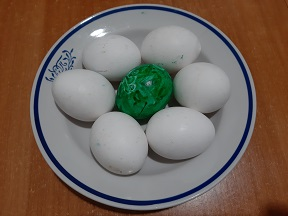Eggs are not 4-dimentional. They are not 2-dimentional objects aither. But we can arrange them across the 2d plane surface.
You, count please, how many white eggs are aroud the central green-painted egg?
Yes!
6!
Of course, in 2d space, or on flat plane surface of your table you can arrange six spheres of exactly the same radius around one central sphere.

We can build from eggs some picturesque 3d constructins.

In 3d space around one sphere we can arrange 12 spheres of exactly the same radius.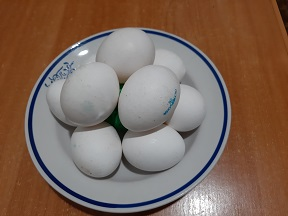You can see the central one, and 9 shell-eggs. Try to put another three eggs on the plate in your imagination. Be carefull and dot´t crash your brain or my eggs! I don´t know the price of your brain but my eggs are very precious.

In 4d space around one four-dimensional sphere we can arrange 24 spheres of the same radius.

On the bottom gif-animated picture you can see the example of the densest packing in the 4d space. For our convenience the spheres are shown with diminished radii, and in the form of circumferences, but not of spheres or circles. That was made in order to see what hides behind the front spheres. The fourth dimension is associated with color. We are situated at the point "green" on the color coordinate. Figuratively speaking, you seat on your chair and you are green in fourth dimension. Your wife can seat on the same chair, if she is red, for example, in fourth dimension. Your girlfriend can also seat on your chair if she is blue. In fact, your single chair can simultaneously house many dozens of your girlfriends if you are a dissolute lecherous man and if they are of different colors.

Well, let’s return to our rotating shitty crappy eggs. The left group of spheres rotates in the horizontal plane xy.
The middle group of spheres rotates in the vertical-color plane zs.
The right group of spheres rotates in both planes, xy and zs simultaneusly.Wow! Four-dimensional rotation has some interesting properties.
1. The right thing is constantly transforms into the left one, and viсe versa. Look! O my God! Right shoe transforms into the left shoe, then into the right again, to the left, right, left......

Figuratively speaking, in order to make the right shoe from the left one, we must turn it in the plane zs on π radians. But if you skipped your geometry classes, and would turn your dirty left hoof around the y axis, you’ll have again the same left hoof....oh my poor dear young idiot! you mixed everything upside down.

So, go and study math.2. These 24 shell-spheres can be pushed into two different rotations simultaneously, and all distances between their centers and the center of the central zero-sphere remain the same! We can see the changing configuration, but those distances do not change. There were no gaps before. There are no gaps between the shell-spheres at any moment of rotation. And everyone of all 24 shell-spheres continues to kiss the central sphere without interruption.

It looks as fantasy, and in reality we cannot make such transformations. But our brilliant egg-headed physicists can make such procedures with elementary particles in CERN tunnels.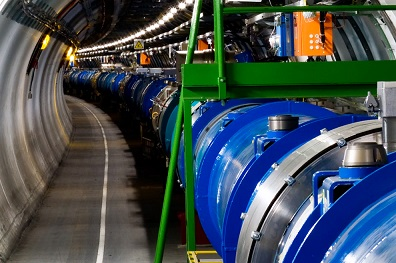It is very dark there in their smelly shitty cellars. There were a lot of rats there. So physicists used the power of large hadron collider in order to collide rats, accelerated to subluminal velocoties. They hoped to receive something new at their 4d portals, which can be openned at such huge energies.

Those high energy rats collisions became the cause of collider crash in 2008.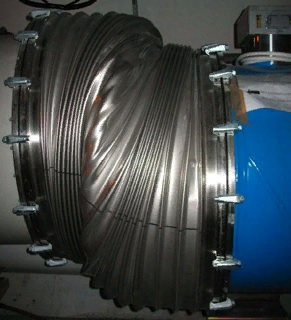Collider had been shut down for about a year. But after the repair, our brilliat scientists resumed their dirty inhumane experiments on the collision of high-energy rats. In 2013, physicists recorded the birth of huge swarms of microscopic red cockroaches, which they passed off as Higgs bosons.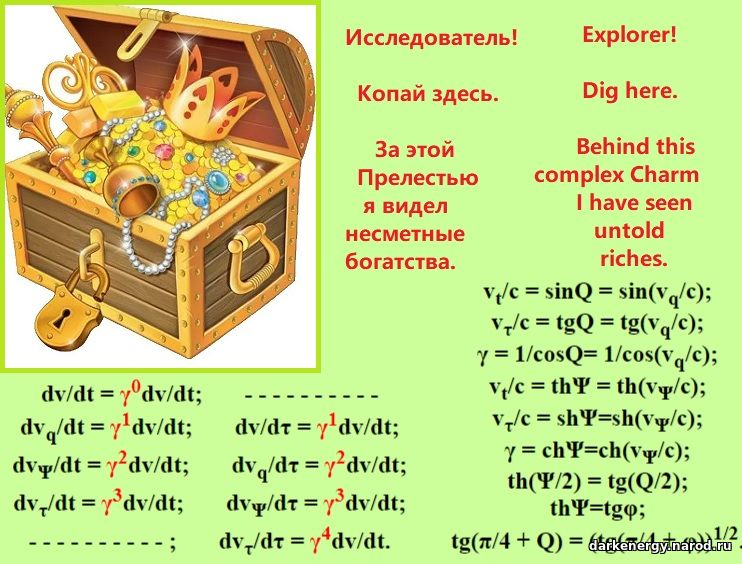Some people believed the egg-headed physicists and bought bottles, filled with invisible microscopic cockroaches, labeled as Higgs bosons. Soon after, a trouble came upon them. Their brains underwent a Big Bang.

Let's leave this sad story and get back to our 4d balls.Well, where are these balls?
OK. I'm not a mathematician. I spent a lot of time and efforts to find the solutions. Then I found them, checked. It came true. Therefore, do not strain your brains - I'll give you the results.

In the bottom text you can see the example set of coordinates of the centers of spheres under the densest packing.
The first six points give us the densest dirty-egg-packing in 2d space;
the first 12 points give us the densest kissing- packing in 3d space;
and at last, all 24 points give us the densest 4d-sphere-packing in 4d space.
Let´s assign the number 0 to the central sphere. Then its coordinates will be four zeroes (0, 0, 0, 0). The spheres, contacting with the central sphere, will receive the numbers-names 1, 2, 3... 24. Coordinates of the spheres are written in the round brackets. The names-numbers of shell-spheres, contacting with the given shell-sphere, is written in the rectangular brackets.

1 (1, 0, 0, 0)      [2,6,7,10,13,16,19,22]
2 (1/2, -√3/2, 0, 0)      [1,3,7,12,15,16,21,22]
3 (-1/2, -√3/2, 0, 0)      [2,4,8,12,15,17,21,23]
4 (-1, 0, 0, 0)      [3,5,8,11,14,17,20,23]
5 (-1/2, √3/2, 0, 0)       [4,6,9,11,14,18,20,24]
6 (1/2, √3/2, 0, 0)         [1,5,9,10,13,18,19,24]
7 (1/2, -1/(2·√3), √2/√3, 0)      [1,2,8,9,13,15,19,21]
8 (-1/2, -1/(2·√3), √2/√3, 0)      [3,4,7,9,14,15,20,21]
9 (0, 1/√3, √2/√3, 0)      [5,6,7,8,13,14,19,20]
10 (1/2, 1/(2·√3), -√2/√3, 0)      [1,6,11,12,16,18,22,24]
11 (-1/2, 1/(2·√3), -√2/√3, 0)      [4,5,10,12,17,18,23,24]
12 (0, -1/√3, -√2/√3, 0)      [2,3,10,11,16,17,22,23]
13 (1/2, 1/(2·√3), 1/√6, 1/√2)      [1,6,7,9,14,15,16,18]
14 (-1/2, 1/(2·√3), 1/√6, 1/√2)      [4,5,8,9,13,15,17,18]
15 (0, -1/√3, 1/√6, 1/√2)       [2,3,7,8,13,14,16,17]
16 (1/2, -1/(2·√3), -1/√6, 1/√2)       [1,2,10,12,13,15,18]
17 (-1/2, -1/(2·√3), -1/√6, 1/√2)       [3,4,11,12,14,15,16,18]
18 (0, 1/√3, -1/√6, 1/√2)       [5,6,10,11,13,14,16,17]
19 (1/2, 1/(2·√3), 1/√6, -1/√2)       [1,6,7,9,20,21,22,24]
20 (-1/2, 1/(2·√3), 1/√6, -1/√2)       [4,5,8,9,19,21,23,24]
21 (0, -1/√3, 1/√6, -1/√2)       [2,3,7,8,19,20,22,23]
22 (1/2, -1/(2·√3), -1/√6, -1/√2)       [1,2,10,12,19,21,23,24]
23 (-1/2, -1/(2·√3), -1/√6, -1/√2)       [3,4,11,12,20,21,22,24]
24 (0, 1/√3, -1/√6, -1/√2)       [5,6,10,11,19,20,22,23]

In order to transit from the turn to rotation, we must express the coordinates with the help of functions sin and cos. Here is the piece of my 25years-old program, which helps us to express the coordinates after every equal interval of time, corresponding to the turn at π/30 radians at both planes of rotation.

fi = pi / 3
M = Sqr(2 / 3)
For t = 0 To 20
psi = 0 + t * pi / 30 'rotation in the plane zc
hi = 0 + t * pi / 30 'rotation in the plane xy
a(0) = 0 'coordinates of the central sphere
b(0) = 0
c(0) = 0
d(0) = 0
For i = 1 To 6 'coordinates of spheres 1, 2,.. 6.
a(i) = Cos(-fi * (i - 1) + hi)
b(i) = Sin(-fi * (i - 1) + hi)
c(i) = 0
d(i) = 0
Next
For i = 7 To 9
a(i) = Cos(-fi / 2 - 2 * fi * (i - 7) + hi) / Sqr(3)
b(i) = Sin(-fi / 2 - 2 * fi * (i - 7) + hi) / Sqr(3)
c(i) = M * Cos(psi)
d(i) = M * Sin(psi)
Next
For i = 10 To 12
a(i) = Cos(fi / 2 + 2 * fi * (i - 10) + hi) / Sqr(3)
b(i) = Sin(fi / 2 + 2 * fi * (i - 10) + hi) / Sqr(3)
c(i) = M * Cos(psi + pi)
d(i) = M * Sin(psi + pi)
Next
For i = 13 To 15
a(i) = -Cos(-fi / 2 - 2 * fi * (i - 13) + hi) / Sqr(3)
b(i) = -Sin(-fi / 2 - 2 * fi * (i - 13) + hi) / Sqr(3)
c(i) = M * Cos(psi + pi / 3)
d(i) = M * Sin(psi + pi / 3)
Next
For i = 16 To 18
a(i) = Cos(-fi / 2 - 2 * fi * (i - 16) + hi) / Sqr(3)
b(i) = Sin(-fi / 2 - 2 * fi * (i - 16) + hi) / Sqr(3)
c(i) = M * Cos(psi + pi + pi / 3)
d(i) = M * Sin(psi + pi + pi / 3)
Next
For i = 19 To 21
a(i) = a(i - 6)
b(i) = b(i - 6)
c(i) = M * Cos(psi + pi + 2 * pi / 3)
d(i) = M * Sin(psi + pi + 2 * pi / 3)
Next
For i = 22 To 24
a(i) = a(i - 6)
b(i) = b(i - 6)
c(i) = M * Cos(psi + 2 * pi / 3)
d(i) = M * Sin(psi + 2 * pi / 3)
Next

At every moment the distance between the central sphere and any of the shell spheres is equal to 1. At every moment the distance between any shell sphere and any of another shell spheres is equal or bigger than 1.
(I don't exclude that this piece of program can be written shorter.)

Earlier I have thought, that in n-dimensional space it is possible to pack m = 3*2n-1 spheres around the central sphere. But it turned out to be, that this formula is correct only for dimensions 2, 3, and 4.

And here is the 2-d rotation of elementary electric charge (electron) in x, ict coordinates, i.e. the rotation in pseudo-Euclidean space-time.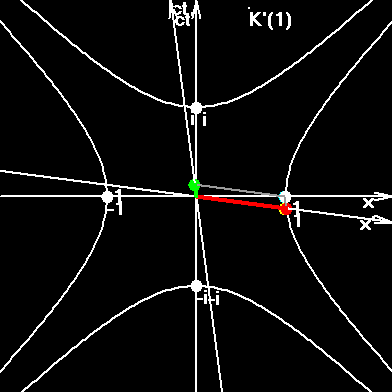## Four-dimensional Rotation of the Universe.

If our Universe is closed, then it can rotate. The last observations give several proves of this idea.

Non-Euclidean closed Universe can be imagined as three-sphere x2+y2+z2 +q2=r2, arranged into Euclidean 4-d space, where q is aditional coordinate, associated with color. That slightly reminds us the left figure, without the central ball. That will be the rotation in 4-d space. But we live in pseudo-Euclidean space-time. That is why, we must change the q-coordinate into ict-coordinate.

In order to imagine the 4d rotation in the pseudo-Euclidean space (r,ict) you must unite both bottom gif-rotaitions in your brain. Try it! It´s easy!It is very interesting that the length of two hyperboles after dividing of every element of hyperboles by corresponding γ is iqual to 2πri. And more, - the quantable velocity was found. As a result the time-axis can by finite and closed.

Now unite the two pictures and imagine the rotation of pseudo-Euclidean hyper-sphere: x2+y2+z2+(ict) 2=r2

All points of the Universe can move with the same linear velocity c, and with the same angular velocity H.

The usual ball can not rotate such, that all it´s point moved with the same linear velocity. In this sense the Universe is more homogeneous.

But we can find one dipole (axis-plane of rotation B) for every point A of such Universe. Moreover any point A has its proper axis-plane B and the point A is situated at B. The point A divides B on to rays. Let say, that this axis-plane is composed by axes z and ict. In every point of the Universe the square of 4-velocity (vγ, icγ), is equal to -c2. At the point A v=0. Consequently its γ=1 and 4-velocyty of this point has only time component (0, ic). Point A moves here with the velocity of light only relatively the time-coordinate. All other points of this axis-plane has a spatial component of 4-velocity. Consequently all galaxies visible from A along the axis-plane (z,ict) will be transverse red-shifted along z-coordinate. But all galaxies laying on this axis-plane does not move relatively each other, - they rotate together along circumference in the plane (z,ict). The plane-axis (x,y), perpendicular to axis (z,ict) is circular and embraces the axis-plane at the distance R. It is also rotating. And the galaxies, visible in that direction will also be transverse red-shifted.

Such rotation explains many-many things, I can not write all here. And only make a note.
Rotation gives and explains:

· the same spin of every corresponding particle of Universe;
· particle´s quantum Ψ-function;
· the right-left asymmetry in galaxies spirality;
· why the conventional age of the Universe is constant and is 13,34 billion years, always!
· abnormal rotational velocities of peripheral stars in galaxies;
· the critical density in such Universe is less...

If the velocity along the axes are slightly different we will see the multipole structure in the cosmic microwave background radiation; the difference in red-shifts of galaxies.

### Kissing Number

 Problems of arranging balls densely arise in many situations, particularly in coding theory (the balls are formed by the sets of inputs that the error-correction would map into a single codeword).
The most important question in this area is Kepler's problem: what is the most dense packing of spheres in space? The answer is obvious to anyone who has seen grapefruit stacked in a grocery store, but a proof remains elusive. (It is known, however, that the usual grapefruit packing is the densest packing in which the sphere centers form a lattice.)

The colorfully named "kissing number problem" refers to the local density of packings: how many balls can touch another ball? This can itself be viewed as a version of Kepler's problem for spherical rather than Euclidean geometry.

 In mathematics, sphere packing problems concern arrangements of non-overlapping identical spheres which fill a space. Usually the space involved is three-dimensional Euclidean space. However, sphere packing problems can be generalised to two dimensional space (where the "spheres" are circles), to n-dimensional space (where the "spheres" are hyperspheres) and to non-Euclidean spaces such as hyperbolic space.
A regular arrangement (also called a periodic or lattice arrangement) is one in which the centres of the spheres form a very symmetric pattern called a lattice. Arrangements in which the spheres are not arranged in a lattice are called irregular or aperiodic arrangements. Regular arrangements are easier to handle than irregular ones—their high degree of symmetry makes it easier to classify them and to measure their densities.

 The number of equivalent hyperspheres in dimensions n which can touch an equivalent hypersphere without any intersections, also sometimes called the Newton number, contact number, coordination number, or ligancy.

Exact values for lattice packings are known for n=1 to 9 and n=24 (Conway and Sloane 1993, Sloane and Nebe). Odlyzko and Sloane (1979) found the exact value for 24-D.

Exact values for general packings are known for n=1, 2, 3, 4, 8, and 24. Musin developed a bounding method in 2003 to prove the 24-dimensional case, and his method also provides proofs for three and four dimensions (Pfender and Ziegler 2004).

### SO(4)

 In mathematics, SO(4) is the four-dimensional rotation group; that is, the group of rotations about a fixed point in four-dimensional Euclidean space. The name comes from the fact that it is (isomorphic to) the special orthogonal group of order 4.

Simple rotations
A simple rotation R about a rotation centre O leaves an entire plane A through O (axis-plane) pointwise invariant...

Half-lines from O in the axis-plane A are not displaced; half-lines from O orthogonal to A are displaced through α; all other half-lines are displaced through an angle < α.

Double rotations
A double rotation R about a rotation centre O leaves only O invariant. Any double rotation has at least one pair of completely orthogonal planes A and B through O that are invariant as a whole, i.e. rotated in themselves. In general the rotation angles α in plane A and β in plane B are different. In that case A and B are the only pair of invariant planes, and half-lines from O in A, B are displaced through α, β, and half-lines from O not in A or B are displaced through angles strictly between α and β.

Isoclinic rotations
If the rotation angles of a double rotation are equal then there are infinitely many invariant planes instead of just two, and all half-lines from O are displaced through the same angle. Such rotations are called isoclinic or equiangular rotations, or Clifford displacements. Beware: not all planes through O are invariant under isoclinic rotations; only planes that are spanned by a half-line and the corresponding displaced half-line are invariant.